Balbharti Maharashtra State Board Class 9 Science Solutions Chapter 1 Laws of Motion Notes, Textbook Exercise Important Questions and Answers.

## Maharashtra State Board Class 9 Science Solutions Chapter 1 Laws of Motion

Class 9 Science Chapter 1 Laws of Motion Textbook Questions and Answers

1. Match the first column with appropriate entries in the second and third columns and remake the table.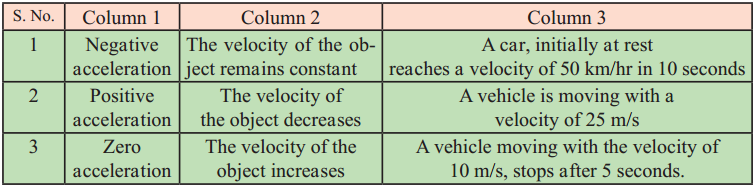2. Clarify the differences

A. Distance and displacement

 Distance Displacement (i) Distance is the length of the actual path travelled by an object. (ii) It is a scalar quantity. (iii) It is either equal to or greater than displacement. (iv) Distance travelled is always positive. (i) Displacement is the minimum distance between the starting and finishing points. (ii) It is a vector quantity. (iii) It is either equal to or less than distance. (iv) Displacement may be positive or negative or zero.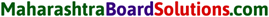B. Uniform and non-uniform motion.

 Uniform motion Non-uniform motion (i) If an object covers equal distances in equal intervals of time it is said to be in uniform motion. (ii) Distance – time graph for uniform motion is a straight line. (iii) In uniform motion, acceleration is zero. (i) If an object moves unequal distances in equal intervals of time, its motion is said to be non­uniform. (ii) Distance – time graph for non-uniform motion is not a straight line. (iii) In non-uniform motion acceleration is non-zero.

3. Complete the following table.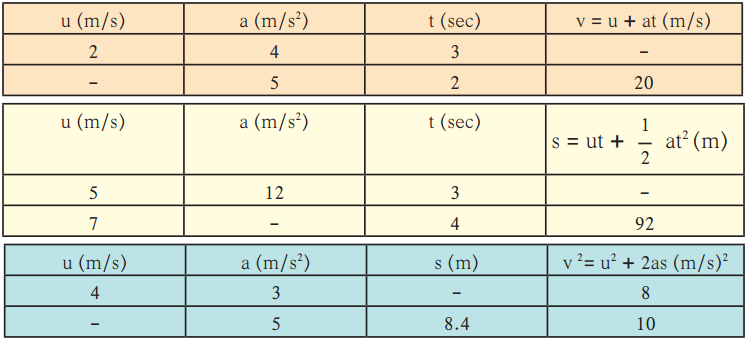4. Complete the sentences and explain them.

a. The minimum distance between the start and finish points of the motion of an object is called the ……….. of the object.
b. Deceleration is ………………………. acceleration
c. When an object is in uniform circular motion, its ………………………. changes at every point.
d. During collision ………………………. remains constant.
e. The working of a rocket depends on Newton’s ………………………. law of motion.

5. Give scientific reasons.

a. When an object falls freely to the ground, its acceleration is uniform.

• When the body falls freely to the ground, there are equal changes in velocity of the body in equal intervals of time.
• Thus the acceleration of the body is constant, and it possesses uniform acceleration.

b. Even though the magnitudes of action force and reaction force are equal and their directions are opposite, their effects do not get cancelled.

• Action and reaction forces act on different bodies.
• They don’t act on the same body, hence they cannot cancel each other’s effect.
• Hence, even though the magnitudes of action force and reaction force are equal, they do not cancel each other.

c. It is easier to stop a tennis ball as compared to a cricket ball, when both are traveling with the same velocity.

• Momentum of an object depends on its mass as well as its velocity.
• Cricket ball is heavier than a tennis ball. Although they are thrown with the same velocity, cricket ball has more momentum than a tennis ball.
• The force required to stop a cricket ball is more than a tennis ball.
• Hence it is easier to stop a tennis ball than a cricket ball moving with same velocity.d. The velocity of an object at rest is considered to be uniform.

• When a body is at rest there is no change in velocity.
• A body with constant velocity is said to be in uniform motion.
• Hence, the state of rest is an example of uniform motion.

6. Take 5 examples from your surroundings and give an explanation based on Newton’s laws of motion.

7. Solve the following examples.

a) An object moves 18 m in the first 3 s, 22 m in the next 3 s and 14 m in the last 3 s. What is its average speed? (Ans: 6 m/s)
Given:
Total distance (d) = 18 + 22 + 14 = 54 m
Total time taken (t) = 3 + 3 + 3 = 9 sec
To find:
Average speed = ?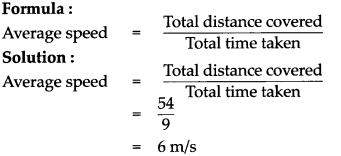The object moves with an average speed of 6 m/s.

b) An object of mass 16 kg is moving with an acceleration of 3 m/s2. Calculate the applied force. If the same force is applied on an object of mass 24 kg, how much will be the acceleration? (Ans: 48 N, 2 m/s2)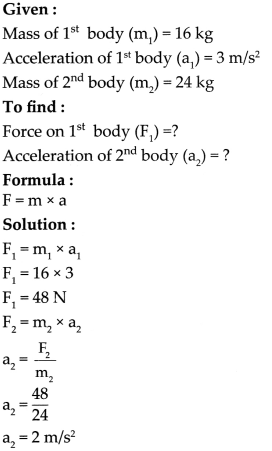The force acting on the 1 body is 48 N and the acceleration of the 2” body is 2 m/s2

c) A bullet having a mass of 10 g and moving with a speed of 1.5 m/s, penetrates a thick wooden plank of mass 90 g. The plank was initially at rest. The bullet gets embedded in the plank and both move together. Determine their velocity. (Ans: 0.15 m/s)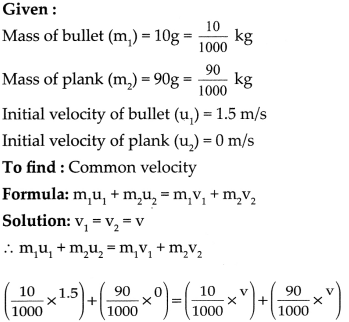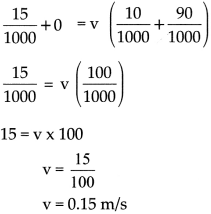The plank embedded with the bullet moves with a velocity of 0.15 m/s.d) A person swims 100 m in the first 40 s, 80 m in the next 40 s and 45 m in the last 20 s. What is the average speed? (Ans: 2.25 m/s2)

Given:
Total distance (d) = 100 + 80 + 45 = 225 m
Total time taken (t) = 40 + 40 + 20 = 100 sec
To find:
Average speed =?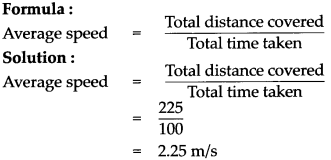The person swims with an average speed of 2.25 m/s.

Class 9 Science Chapter 1 Laws of Motion Intext Questions and Answers

(i) Who will take less time to reach the school and why?
Prashant will take less time as the path followed by him is the shortest.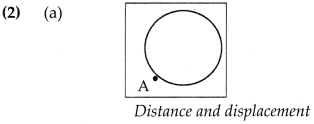(a) Every morning, Swaralee walks round the edge of a circular field having a radius of 100 m. As shown in figure (a), if she starts from the point A and takes one round, how much distance has she walked and what is her displacement?
Distance covered = Circumference of the circle
= 2 nr
= 2 x 3.14 x 100
= 628 m
Displacement = 0 m (Shortest distance between initial and final position is zero)

(b) If a car, starting from point P, goes to point Q (see figure 1.9) and then returns to point P, how much distance has it travelled and what is its displacement?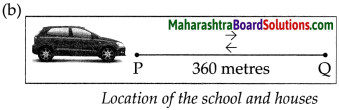Distance covered = PQ + QP
= 360 + 360
= 720 m
Displacement = 0 m (The shortest distance between initial and final position is zero)

Class 9 Science Chapter 1 Laws of Motion Additional Important Questions and Answers

(A) Choose and write the correct option:

Laws Of Motion Class 9 Questions And Answers Maharashtra Board Question 1.
The displacement that occurs in unit time is called …………….. .
(a) displacement
(b) distance
(c) velocity
(d) acceleration
(c) velocityLaws Of Motion Class 9 Maharashtra Board Exercise Answers  Question 2
The unit of velocity in the SI system is …………….. .
(a) cm/s
(b) rn/s2
(c) um/s2
(d) rn/s
(d) m/s

Laws Of Motion Class 9 Maharashtra Board  Question 3.
v2 = u2 + 2as is the relation between and …………….. .
(a) speed and velocity
(b) distance and acceleration
(c) displacement and velocity
(d) speed and distance
(c) displacement and velocity

Class 9 Science Notes Chapter 1 Laws Of Motion Question 4.
…………….. is the relation between displacement and time.
(a) v = u + at
(b) v2 = u2 + 2as
(c) s = ut + 1/2 at2
(d) v = u + 2as
(c) s = ut + 1/2 at2

Class 9 Science Chapter 1 Laws Of Motion Question Answer Question 5.
The force necessary to cause an acceleration of 1 m/s2 in an object of mass 1 kg is called …………….. .
(a) 1 dyne
(b) 1 m/s
(c) 1 Newton
(d) 1 cm/s
(c) 1 Newton.

9th Science Chapter 1 Laws Of Motion Question 6.
Even if the displacement of an object is zero, the actual distance traversed by it …………….. .
(a) may not be zero.
(b) will be zero
(c) will be constant
(d) will be infinity
(a) may not be zero

Question 7.
If the velocity changes by equal amounts in equal time intervals, the object is said to be in …………….. .
(a) uniform acceleration
(b) uniform velocity
(c) non-uniform acceleration
(d) non-uniform motion
(a) uniform acceleration

Question 8.
If an object is moving with a uniform velocity …………….. .
(a) its speed remains the same, but direction of motion changes
(b) its speed changes but direction of motion is same
(c) its speed and direction both change
(d) its speed and direction both remain the same
(d) its speed and direction both remain the same

Question 9.
is an example of positive acceleration.
(a) A stone is thrown vertically upwards
(b) A stone falls freely towards the earth
(c) Brakes are applied by the truck driver
(d) The train arriving at the station
(b) a stone falls freely towards the earthQuestion 10.
An object continues to remain at rest or in a state of uniform motion along a straight line unless an …………….. acts on it.
(a) internal imbalanced force
(b) external unbalanced force
(c) internal balanced force
(d) external balanced force
(b) external unbalanced force

Question 11.
The …………….. is proportional to the applied force and it occurs in the direction of the force.
(a) change of momentum
(b) rate of change of velocity
(c) change of velocity
(d) rate of change of momentum
(d) rate of change of momentum

Question 12.
…………….. is a relative concept.
(a) Motion
(b) Direction
(c) Power
(d) Acceleration
(a) Motion

Question 13.
A body is said to be in motion if it changes its …………….. with respect to its surroundings.
(a) position
(b) direction
(c) speed
(d) force
(a) position

Question 14.
A body is said to be at …………….. if it does not change its position with respect to its surroundings.
(a) Motion
(b) Rest
(c) Gravity
(d) Force
(b) Rest

Question 15.
…………….. is the length of the actual path travelled by an object in motion while going from one point to another.
(a) Distance
(b) Displacement
(c) Speed
(d) Velocity
(a) Distance

Question 16.
The distance covered by a body in unit time is called its …………….. .
(a) velocity
(b) speed
(c) displacement
(d) rest
(b) speedQuestion 17.
S.I. unit of speed is …………….. and in C.G.S unit it is …………….. .
(a) m/s and cm/s
(b) km/s and cm/s
(c) m/s and mm/s
(d) m/s and nm/s
(a) m/s , cm/s

Question 18.
The distance travelled in a particular direction by an object in unit time is called its …………….. .
(a) velocity
(b) speed
(c) displacement
(d) rest
(a) velocity

Question 19.
Units of speed and velocity are the
(a) Same
(b) Different
(c) Greater than each other
(d) Unequal
(a) same

Question 20.
…………….. . is related to distance, while …………….. is related to displacement.
(a) Gravity and magnetism
(b) Speed and force
(c) Speed and velocity
(d) Motion and rest
(c) Speed, velocity

Question 21.
If an object covers equal distances in equal time intervals, it is said to be moving with …………….. speed.
(a) Uniform
(b) Non uniform
(c) Changing
(d) Random
(a) uniform

Question 22.
If an object covers unequal distances in equal time Intervals, it is said to be moving with speed.
(a) Uniform
(b) Non uniform
(c) Changing
(d) Random
(b) non uniformQuestion 23.
The rate of change of velocity is called
(a) Speed
(b) Acceleration
(c) Velocity
(d) Rest
(b) acceleration

Question 24.
Speed of light in dry air is …………….. m/s.
(a) 3 x 107
(b) 3 x 108
(c) 3 x 109
(d) 3 x 103
(b) 3 x 108

Question 25.
When velocity of a body increases, its acceleration is …………….. .
(a) Negative
(b) Zero
(c) Positive
(d) Equal
(c) positive

Question 26.
When velocity of a body decreases, its acceleration is …………….. .
(a) Negative
(b) Zero
(c) Positive
(d) Equal
(a) negative

Question 27.
Negative acceleration is also called or
(a) Deceleration or retardation
(b) Deceleration or acceleration
(c) acceleration or retardation
(d) Zero
(a) deceleration or retardation

Question 28.
In case of motion, object travels equal …………….. in equal intervals of time.
(a) Uniform, distance
(b) Non-Uniform, distance
(c) Uniform, displacement
(d) Uniform, displacement
(a) uniform, distancesQuestion 29.
Motion of an object was studied by …………….. .
(a) Sir Albert Einstein
(b) Sir Thomas Edison
(c) Sir Isaac Newton
(d) Sir Ravindranath Tagore
(c) Sir Issac Newton

Question 30.
When an object moves in a circular path with uniform speed, its motion is …………….. motion.
(a) Non uniform circular
(b) Random circular
(c) Uniform circular
(d) Uniform linear
(c) uniform circular

Question 31.
When a coin moves along a circular path, the direction of its motion at every point is …………….. .
(a) Circular
(b) Concave
(c) Tangential
(d) Convex
(c) tangential

Question 32.
For all uniformly accelerated motions, the velocity-time graph is a …………….. .
(a) Curved line
(b) Straight line
(c) Negative line
(d) Positive line
(b) straight line

Question 33.
In the distance-time graph, the slope of the straight line indicates …………….. .
(a) Acceleration
(b) Velocity
(c) Speed
(d) Rest
(b) velocity

Question 34.
The first equation of motion gives relation between …………….. and time.
(a) Acceleration
(b) Velocity
(c) Speed
(d) Rest
(b) velocityQuestion 35.
Newton’s first law explains the phenomenon of
(a) Rest
(b) Inertia
(c) Speed
(d) Velocity
(b) inertia

Question 36.
…………….. cause a change in the state of an object at rest or in uniform motion.
(a) Balanced forces
(b) Zero forces
(c) Unbalanced forces
(d) None of them
(c) Unbalanced forces

Question 37.
To describe an object’s momentum, we must specify its …………….. and …………….. .
(a) Mass and displacement
(b) Mass and direction
(c) Mass and velocity
(d) Mass and acceleration
(c) mass and velocity

Question 38.
…………….. is the product of mass and velocity of an object.
(a) Speed
(b) Acceleration
(c) Momentum
(d) Force
(c) Momentum

Question 39.
The rate of change of momentum is proportional to the applied …………….. .
(a) Balanced force
(b) Unbalanced force
(c) Mass
(d) Velocity
(b) unbalanced force

Question 40.
S.I. unit of momentum is
(a) kg cm/s
(b) kg m/s
(c) gm/s
(d) m/s
(b) kg m/sQuestion 41.
…………….. is always conserved in a collision.
(a) Force
(b) Power
(c) Speed
(d) Total momentum
(d) Total momentum

Question 42.
When a bullet is fired from the gun, the gun moves in backward direction. This motion is called as …………….. .
(a) Momentum
(b) Velocity
(c) Acceleration
(d) Recoil
(d) Recoil

Question 43.
In CGS system, the unit of force is …………….. .
(а) Newton
(b) Watt
(c) Horse power
(d) Dyne
(d) Dyne.

(B) 1. Find the odd man out:

Question 1.
Displacement, Force, Momentum, Mass
Mass

Question 2.
Speed, Power, Energy, Acceleration
Acceleration

Question 3.
Newton’s 1st law, Newton’s 2nd law, Newton’s 3rd law, Kepler’s laws of motion
Newton’s 3rd law

(B) 2. Find out the correlation

Question 1.
Speed zero: Body at rest :: Negative acceleration : Retardation
RetardationQuestion 2.
Displacement : Vector quantity :: Distance : Scalar quantity
Vector quantity

Question 3.
When body comes to rest at the end of the motion : Final velocity is zero :: When body is at rest at the starting of motion : Initial velocity is zero
Initial velocity is zero

Question 4.
Uniform circular motion: Displacement is zero :: Uniform velocity : Acceleration is zero
Displacement is zero

Question 5.
Inertia : Newton’s 1st law :: Rate of change of momentum : Newton’s 2nd law
Newton’s 2nd law

Question 6.
Balanced force : Body at rest :: Force equation : Mass x acceleration
Body at rest

(B) 3. Distinguish between:

Question 1.
Positive acceleration and Negative acceleration

 Positive acceleration Negative acceleration (i) When the velocity of a body increases, acceleration is said to be positive acceleration. (i) When the velocity of a body decreases, acceleration is said to be negative acceleration.

Question 2.
Scalar quantity and Vector quantity

 Scalar quantity Vector quantity (i) Scalar quantities are physical quantities having magnitude only. (i) Vector quantities are physical quantities having both magnitude and direction.Question 3.
Balanced force and Unbalanced force

 Balanced force Unbalanced force (i) Balanced force keeps the body at rest. (i) Balanced force keeps the body at rest.

(B) 4. State whether the following statements are true or false:

Question 1.
The velocity of a body is given by the distance covered by it in unit time in a given direction.
True

Question 2.
Displacement is a scalar quantity.
False

Question 3.
Uniform acceleration means that the body is moving with a uniform velocity.
False

Question 4.
The direction of acceleration can be opposite to that of velocity.
True

Question 5.
Work is a vector quantity.
False

Question 6.
Displacement is always greater than distance.
FalseQuestion 7.
The distance and displacement are equal only if, motion is along a straight path.
True

Question 8.
If an object experiences acceleration, a force is acting on it.
True

Question 9.
A train pulling out from a station is in uniform motion.
False

Question 10.
If a bus in motion is suddenly stopped, the passengers fall backwards.
False

Question 11.
If a single force is acting on an object, it will always accelerate.
True

Question 12.
In circular motion, direction of motion is tangential.
True

Question 13.
The inertia of a body is measured in terms of its mass.
True

(B) 5. Name the following:

Question 1.
The scientist who summarized motion in a set of equations of motion.
Isaac Newton

Question 2.
Motion of an object along a circular path with uniform speed.
Uniform circular motionQuestion 3.
What is the backward motion of the gun called?
Recoil

Question 4.
The motion in which the object covers equal distance in equal intervals of time.
Uniform motion

Question 5.
S. I. unit of acceleration.
m/s2

Question 6.
CGS unit of momentum
g cm/s

(B) 6. Answer the following in one sentence:

Question 1.
When is acceleration said to be positive?
When the velocity of a body increases, acceleration is said to be positive acceleration.

Question 2.
What is negative acceleration?
When the velocity of a body decreases, acceleration is said to be negative acceleration.

Question 3.
What is the direction of velocity of an object performing uniform circular motion?
The direction of velocity is along the tangential direction to its position.

Question 4.
Give the mathematical expression used to determine velocity of an object moving with uniform circular motion.
is the expression used to determine velocity of a body moving with uniform circular motion.

Question 5.
What kind of force keeps the body at rest?
Balanced force keeps the body at rest.Question 6.
Which law of motion gives the measure of force?
Newton’s second law of motion gives the measure of force.

Question 7.
What are vectors and scalars?
Scalars are physical quantities having magnitude only whereas, vectors are physical quantities having both magnitude and direction.

Question 8.
Which of the quantities distance, speed, velocity, time and displacement are scalars and which are vectors?
Distance, speed and time are scalars displacement and velocity are vectors.

Give formula:

Question 1.
Force =
Mass x Acceleration = ma

Question 2.
Final velocity (v) =
Initial Velocity + (Acceleration x Time) = u + at

Question 3.
Displacement (s) =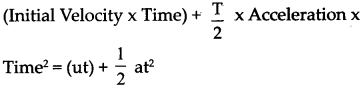Question 4.
Final velocity2 (v2) =
Initial Velocity2 + 2 x Acceleration x Displacement = u2 + 2as

Question 5.
velocity of an object moving with uniform circular motion =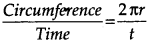Give scientific reasons:

Question 5.
Motion is relative.

• The motion of an object depends on the observer, hence a body may appear moving for one person and at the same time at rest for another one.
• Hence, motion is relative.Question 6.
Newton’s first law of motion is called as law of inertia.
OR
Heavier objects offer more inertia.

• Inertia is related to the mass of the object.
• As mass is the quantity of matter in a body, we need to exert more force to push a heavier body.
• Hence heavier objects offer more inertia.
• As the same property is described by Newton’s first law of motion, it is called as Law of Inertia.

Question 7.
The launching of a rocket is based on Newton’s third law of motion.

• Newton’s third law of motion states that ‘Every action force has an equal and opposite reaction force which acts simultaneously.’
• When the fuel in a rocket is ignited, it bums as a result of chemical reaction.
• The exhaust gases escape with a great force in the backward direction.
• It exerts an equal and opposite reaction force on the rocket, due to which the rocket moves in the forward direction.
• Thus, the principle of launching of rocket is based on Newton’s third law of motion.

Solve the following numerical:

Question 1.
An athlete is running on a circular track. He runs a distance of 400 m in 25 s before returning to his original position. What is his average speed and velocity?
Given:
Total distance travelled = 400 m
Total displacement = 0, as he returns to his original position.
Total time = 25 seconds.
To find:
Average speed = ?
Average velocity = ?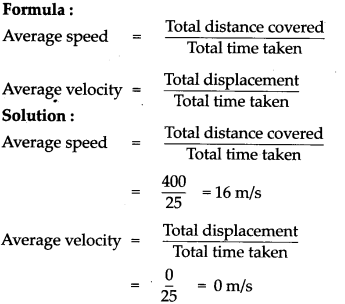The athlete runs at an average speed of 16 m/s and velocity 0 m/s.Question 2.
An aeroplane taxies on the runway for 30 s with an acceleration of 3.2 m/s2 before taking off. How much distance would it have covered on the runway?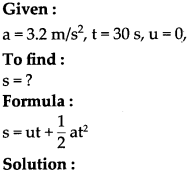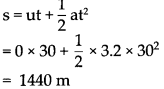The distance covered on the runway is 1440 m.

Question 3.
A kangaroo can jump 2.5 m vertically. What must be the initial velocity of the kangaroo?
Given:
a = 9.8 m/s2, s = 2.5 m, v = 0,
To find:
u = ?
Formula:
v2 = u2 + 2as
Solution:
v2 = u2 + 2as
02 = u2 + 2 x (-9.8) (2.5) : (Negative sign is used as the acceleration is in the direction opposite to that of velocity.)
0= u2 – 49 u2
= 49 u
= 7 m/s

The initial velocity of the kangaroo must be 7 m/s.

Question 4.
A motorboat starts from rest and moves with uniform acceleration, if it attains the velocity of 15 m/s in 5s, calculate the acceleration and the distance travelled in that time.
Given:
Initial velocity, u = 0
Final velocity, v = 15 m/s time, t = 5 s.
To find:
Acceleration (a) = ?
Distance (s) = ?
Solution:
From the first equation of motion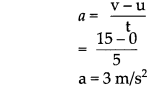From the second equation of motion, the distance covered will be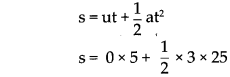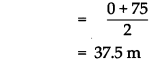The acceleration is 3 m/s2 and distance travelled is 37.5 m.Question 5.
The mass of a cannon is 500 kg and it recoils with a speed of 0.25 m/s. What is the momentum of the cannon?
Given:
mass of the cannon = 50 kg
recoil speed = 0.25 m/s
To find:
Momentum = ?
Formula:
Momentum = m x v
Solution:
Momentum = m x v
= 500 x 0.25
= 125 kg m/s

The momentum of cannon is 125 kg m/s

Question 1.
Explain the three different ways to change the velocity.
As velocity is related to speed and direction, it can be changed by :

• Changing the speed while keeping the direction constant.
• Changing direction while keeping speed constant.
• Changing both speed as well as direction of motion.

Question 2.
Explain what is positive, negative and zero acceleration.

• Positive Acceleration: When the velocity of an object increases, the acceleration is positive. In this case, the acceleration is in the direction of velocity.
• Negative Acceleration: When the velocity of an object decreases with time, it has negative acceleration. Negative acceleration is also called deceleration. Its direction is opposite to the direction of velocity.
• Zero Acceleration: If the velocity of the object does not change with time, it has zero acceleration.

Question 3.
What inference do we draw from the velocity-time graph for a uniformly accelerated motion?

• From velocity-time graph we can infer whether velocity changes by equal amounts in equal intervals of time or not.
• Thus, for all uniformly accelerated motion, the velocity – time graph is a straight line and slope of the line gives the acceleration.
• For non-uniformly accelerated motion, velocity-time graph can have any shape according to variation in velocity with respect to time.Question 4.
State the three equations of motion and give the relationship explained by them.

• v = u + at: This is the relation between velocity and time.
• s = ut + 1/2 at2 : This is the relation between displacement and time
• v2 = u2 + 2as : This is the relation between displacement and velocity.

Question 5.
What are the implications of Newton’s Third Law of motion?

• Action and reaction are terms that express force.
• These forces act in pairs. One force cannot exist by itself.
• Action and reaction forces act simultaneously.
• Action and reaction forces act on different objects. They do not act on the same object and hence cannot cancel each other’s effect.

Question 6.
Explain recoil and recoil velocity. Derive its expression.

• Let us consider the example of a bullet fired from a gun. When a bullet of mass nij is fired from a gun of mass m2, its velocity becomes v2, and its momentum becomes m^. Before firing the bullet, both the gun and the bullet are at rest. Hence, total initial momentum is zero.
• According to Newton’s third law of motion, the total final momentum also has to be zero. Thus, the forward-moving bullet causes the gun to move backward after firing.
• This backward motion of the gun is called its recoil. The velocity of recoil, v2 is such that,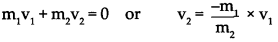Complete the flow chart:

(1) Types of force and their effects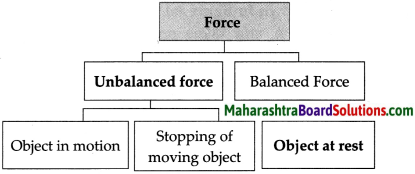(2) Newton’s laws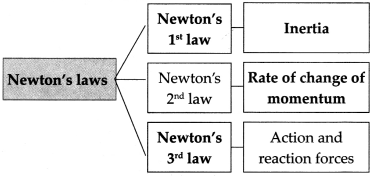Distinguish between:

Question 1.
Speed and velocity.

 Speed Velocity (i) Speed is the distance covered by a body in unit time. (ii) It is a scalar quantity. (iii) Speed is equal to or greater than velocity. (iv) Speed = distance/time (v) It is always positive or zero but never negative. (i) The displacement that occurs in unit time is called velocity. (ii) It is a vector quantity. (iii) Velocity is equal to or less than speed. (iv) Velocity = displacement/time (v) It may be positive, Zero or negative.Question 2.
Balanced force and unbalanced force.

 Balanced force Unbalanced force (i) Two equal forces applied on a body in the opposite direction. (ii) This force does not change the state of rest or the state of uniform motion (i) Two unequal forces applied on a body. (ii) This force can change the state of rest or the state of uniform motion of a body in a straight line.

Give examples:

Question 1.
Scalar quantities
Time, Volume, Speed, Mass, Temperature, Distance, Entropy, Energy, and Work

Question 2.
Vector quantities
Acceleration, Velocity, Momentum, Force, and Weight

Observe the figure and answer the questions: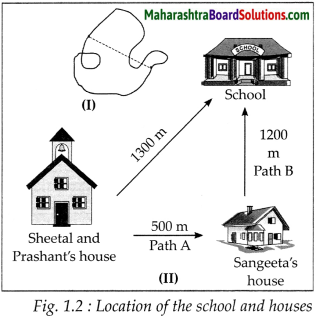(a) Measure the distance between points A and B in different ways as shown in figure (I).
Distances measured may be of different lengths depending on the path taken.

(b) Now measure the distance along the dotted line. Which distance is correct according to you and why?
Dotted line shows the shortest way of reaching from A to B.(c) Observe the following figures. If you increase the number of sides of the polygon and make it infinite, how many times will you have to change the direction? What will be the shape of the path?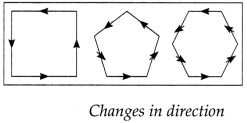If we increase the number of sides of the polygon and make it infinite, then we will have to change the direction an infinite number of times. The shape of the path thus obtained will be a circle.

Observe the figure and answer the questions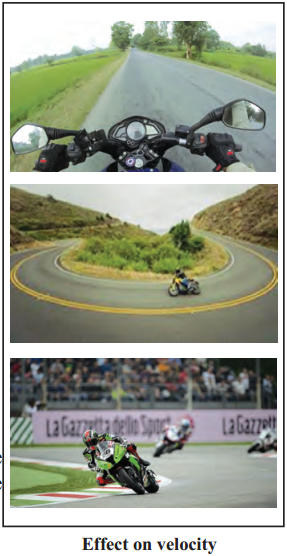Question 1.
What will be the effect on the velocity of the motorcycle if its speed increases or decreases, but its direction remains unchanged?
If the speed is increased the velocity of the motorcycle will increase and if the speed is decreased the velocity of the motorcycle will decrease

Question 2.
In case of a turning on the road, will the velocity and speed be same?
As speed is scalar quantity while velocity is vector quantity so by turning velocity will change while speed remains same

Question 3.
If, on a turning, we change the direction as well as the speed of the motorcycle, what will be the effect on its velocity?
Its velocity will change because velocity depends on speed as well as direction and here both speed and direction are changed.Numerical:

Question 1.
A person travels a distance of 72 km in 4 hours. Calculate average speed in m/s.
Given :
Total distance (d)
= 72 km
= 72×1000
= 72000 m

Total time taken (t)
= 4 hours
= 4 x 3600 (v lhr = 3600 sec)
= 14400 s

To find:
Average speed = ?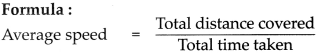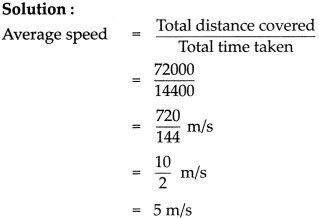The person travels with average speed of 5 m/s

Question 2.
balls have masses of 50 gm and 100 gm and they are moving along the same line in the same direction with velocities of 3 m/s and 1.5 m/s respectively. They collide with each other and after the collision, the first ball moves with a velocity of 2.5 m/s. Calculate the velocity of the other ball after collision.Final velocity of second ball after collision is 1.75 m/s.Write laws and explain write implications:

Question 1.
Newton’s third law of motion
‘Every action force has an equal and opposite reaction force which acts simultaneously’.

• Action and reaction are terms that express force.
• These forces act in pairs. One force cannot exist by itself.
• Action and reaction forces act simultaneously.
• Action and reaction forces act on different objects. They do not act on the same object and hence cannot cancel each other’s effect.

Question 2.
Explain Newton’s second law of motion and derive the formula.
Newton’s second law explains about change in momentum. It states that The rate of change of momentum is proportional to the applied force and the change of momentum occurs in the direction of the force.

Suppose an object of mass ‘m’ has an initial velocity ‘u. When a force ‘F’ is applied in direction of its velocity for time ‘t’, its velocity becomes ‘y’. Then, the total initial momentum of the body = ‘mu’. Its final momentum after time t = ‘mv’.

So, the rate of change of momentum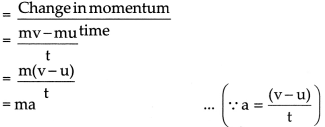Hence by Newtons second la of motion, 4he rate of change of momentum is proportional to the applied force.
∴ ma ∝ F
∴ F ∝ ma
∴ F ∝ kma (k = Constant of proportionaLity and value is 1).
∴ F = maQuestion 3.
State the law of conservation of momentum and derive the formula.

Let mass of object A and B be m1 and m1 respectively
Let their initial velocity be u1 and u2 Let their final velocity be v1 and v2

We know,
P = mv
Let their initial momentum be m1u1 and m2 u2
Let their final momentum be m1v1 and m2v2

Total initial momentum = (m1u1 + m2u2)
Total final momentum = (m1v1 + m2v2)

If F2 is the force that acts on object B,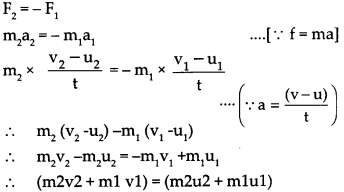i.e. The magnitude of total of total final momentum = the magnitude of total initial momentum

Complete the paragraph:

Question 1.
Moving Objects
‘Distance’ is the length of the actual path travelled by an object in motion while going from one point to another, whereas displacement is the minimum distance between the starting and finishing points. The displacement that occurs in unit time is called velocity. The units of speed and velocity are the same. In the SI system, the unit is m/s while in the CGS system; it is cm/s. Speed is related to distance while velocity is related to the displacement. If the motion is along a straight line, the values of speed and velocity are the same, otherwise they can be different. The first scientist to measure speed as the distance /time was Galileo. The speed of sound in dry air is 343.2 m/s while the speed of light is about 3 x 108 m/s. The speed of revolution of the earth around the sun is about 29770 m/s.

Question 2.
Types of motion
If an object covers unequal distances in equal time intervals, it is said to be moving with non-uniform speed. For example the motion of a vehicle being driven through heavy traffic. If an object covers equal distances in equal time intervals, it is said to be moving with uniform speed. The rate of change of velocity is called acceleration. If the velocity changes by equal amounts in equal time intervals, the object is said to be in uniform acceleration. If the velocity changes by unequal amounts in equal time intervals, the object are said to be non-uniform acceleration. The speed of the tip is constant, but the direction of its displacement and therefore, its velocity is constantly changing. As the tip is moving along a circular path, its motion is called uniform circular motion.Question 3.
Newton’s laws and conservation of momentum
An object continues to remain at rest or in a state of uniform motion along a straight line unless an external unbalanced force acts on it. The rate of change of momentum is proportional to the applied force and the change of momentum occurs in the direction of the force. Momentum has magnitude as well as direction. Its direction is the same as that of velocity. In SI system, the unit of momentum is kg m/s, while in CGS system, it is g cm/s. If an unbalanced force applied on an object causes a change in the velocity of the object, then it also causes a change in its momentum.

The force necessary to cause a change in the momentum of an object depends upon the rate of change of momentum. Every action force has an equal and opposite reaction force which acts simultaneously. As the mass of the gun is much higher than the mass of the bullet, the velocity of the gun is much smaller than the velocity of the bullet. The magnitude of the momentum of the bullet and that of the gun are equal and their directions are opposite. Thus, the total momentum is constant. Total momentum is also constant during the launch of a rocket.

Question 1.
What is speed? State its units and types. Explain instantaneous speed and average speed.

The speed of a body is the distance travelled in unit time. The units of speed in CGS system is cm/s and in SI system is m/s.
There are two types of speed :

• Uniform speed : When a body covers equal distance in equal intervals of time throughout its motion, it is said to have uniform speed.
• Non-uniform or variable speed: When a body covers unequal distance in equal intervals of time it is said to have non-uniform speed.

The speed of the body at any instant is called instantaneous speed. Average speed is the ratio of total distance covered to total time taken.

Question 2.
What is velocity? State its units and types.

The velocity of a body is the distance travelled by a body in a particular direction in unit time. Thus, rate of change of displacement is called velocity.

v = s/t
where: s = displacement; t = time taken; v = velocity
(MKS unit: m/s CGS unit: cm/s)
There are two types of velocities :

• Uniform velocity: If there is equal displacement taking place in equal intervals of time, it is uniform velocity.
• Non-uniform velocity or variable velocity: If there is unequal displacement in equal intervals of time, it is non-uniform velocity.Question 3.
What is acceleration? State its units and types.
(i) Acceleration is a rate of change in velocity. It is a vector quantity, $$a=\frac{v-u}{t}$$
where : v = final velocity; u = initial velocity;
a = acceleration
Units of acceleration in SI system is m/s2 and CGS system is cm/s2.

(ii) Types of acceleration: .
(a) Uniform acceleration : If the change in velocity is equal in equal intervals of time, the acceleration is uniform acceleration.
(b) Non-uniform acceleration : If the change in velocity is unequal in equal intervals of time, the acceleration is a non-uniform acceleration.

(iii) Kinds of acceleration:
Positive acceleration : When the velocity of an object goes on increasing, it is said to have Positive acceleration.
Negative acceleration : When the velocity of an object goes on decreasing, it is said to have negative acceleration or retardation or deceleration.
Zero acceleration : If the velocity of the object does not change with time, it has zero acceleration.

Question 4.
Explain Newton’s second law of motion and derive the formula.
Newton’s second law explains about change in momentum. It states that ‘The rate of change of momentum is proportional to the applied force and the change of momentum occurs in the direction of the force.’

Suppose an object of mass’m’ has an initial velocity ‘u’. When a force ‘F’ is applied in the direction of its velocity for time’t’, its velocity becomes ‘v’. Then, the total initial momentum of the body = ‘mu’. Its final momentum after time t = ‘mv’.

So, the rate of change of momentum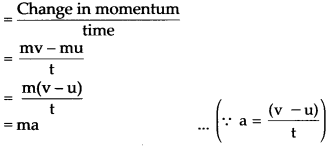Hence by Newton’s second law of motion, the rate of change of momentum is proportional to the applied force.
∴ ma ∝ F
∴ F ∝ ma
∴ F = kma (k = Constant of proportionality and value is 1).
∴ F = maQuestion 5.
State the law of conservation of momentum and derive the formula.
(i) Let mass of objects A and B be and m2 respectively
Let their initial velocity be m1 and u2
Let their final velocity be V1 and v2

(ii) We know,
P = mv
Let their initial momentum be m1u1 and m2u2
Let their final momentum be m1v1 and m2v2

(iii) Total initial momentum = (m1u1 + m2u2)
Total final momentum = (m1v1 + m2v2)

(iv) If F2 is the force that acts on object B,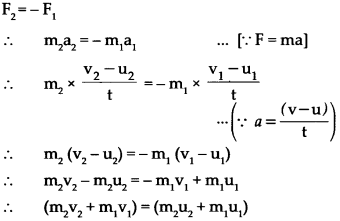i.e. The magnitude of total final momentum = the magnitude of total initial momentum.

Question 6.
Obtain the equations of motion by graphical method:

(a) Equation for velocity-time relation.
Velocity-time graph: shows the change in velocity with time of a uniformly accelerated object. The object starts from the point D in the graph with velocity v. Its velocity keeps increasing and after time t it reaches the point B on the graph.

The initial velocity of the object = u = OD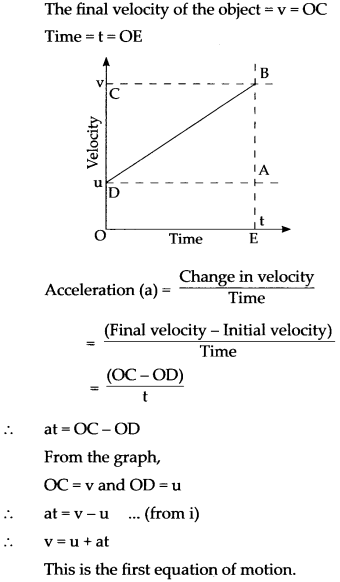(b) Equation for displacement-time relation.
Suppose that an object is in uniform acceleration ‘a’ and it has covered the distance ‘s’ within time’t’. From the graph the distance covered by the object during time’t’ is given by the area of quadrangle DOEB.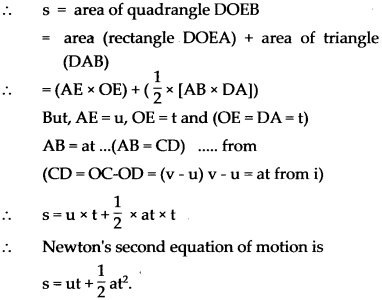(c) Equation for displacement-velocity relation.
We can determine the distance covered by the object in time t from the area of the quadrangle DOEB. DOEB is a trapezium. So we can use the formula for its area.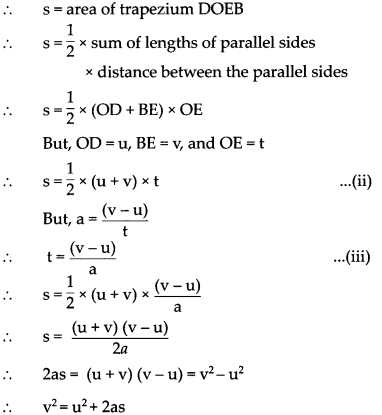This is Newton’s third equation of motion.

1. Paragraph

(a) When a bat strikes a ball, the ball exerts an equal and opposite force on the bat. The force : acting on the ball projects it with high velocity, Due to the large mass of bat compared to ball, reaction force on the bat slows down the bat’s motion.
(b) When a bullet is fired from a gun, the gun exerts a force on the bullet in the forward direction. This is the action force. The bullet also exerts an equal force on the gun in the backward direction. This is the reaction force. Due to the large mass of the gun, it j moves only a little distance backward. This backward movement of the gun is called the recoil of the gun.
(c) In a rocket, burning fuel creates a push on the front of the rocket pushing it forward. This creates an equal and opposite push on the exhaust gas backwards.(i) Which of Newton’s law examples are given here?
Newton’s 3rd law is stated by the above example

(ii) When a rifle is fired it is pushed back this movement is called what?
Movement of rifle getting pushed back after firing is called recoil.

(iii) What does the ball acquire after it gets velocity?
The ball acquires momentum after it gets velocity.

(iv) State newton’s 1st law of motion
An object continues to remain at rest or in a state of uniform motion along a straight line unless an external unbalanced force acts on it.

(v) Which force is required to produce motion in an object?
Unbalanced force is required to produce motion in an object.

2. Paragraph 2
constant velocity. If that velocity is zero, then the object remains at rest. If an external force is applied, the velocity will change because of the force. The second law explains how the velocity of an object changes when it is subjected to an external force. The law defines a force to be equal to changes in momentum (mass times velocity) per change in time. Newton also developed the calculus of mathematics, and the “changes” “expressed in j the second law are most accurately defined in differential forms. (Calculus can also be used to determine the velocity and location variations experienced by an object subjected to an external force.) For an object with a constant mass the second law states that the force F is the product of an objects mass and its acceleration a:
F = m * a
For an external applied force the change in velocity depends on the mass of the object.

A force will cause in velocity; and likewise, a change in velocity will generate a force. The equation works both ways.
The third law states that for every action (force) in nature there is an equal and opposite reaction. In other words, if object A exerts a force on object B, then object B also exerts an equal force on object: A. Notice that the forces are exerted on different objects. The third law can be used to explain the generation of lift by a wing and the production of thrust by a jet engine

(i) A chalk kept on the table remains in the position of rest until moved by the teacher. Which law of motion is followed in this situation?
Newton’s 1st law is followed in this situation e

(ii) What will happen to momentum if the mass and acceleration both are doubled?
If the mass and acceleration both are doubled then the momentum will be increased four times

(iii) What will happen to momentum if the mass and acceleration both are halved?